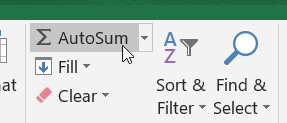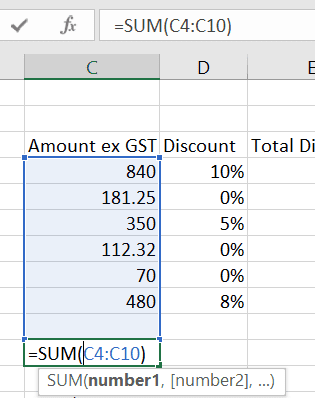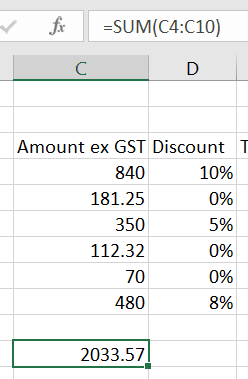Home » Our Blog » How to total a range of cells in Excel

How to total a range of cells in ExcelThe quickest way to total a range of cells in Excel is to use the SUM function.

The Sum function is one of the most commonly used functions in Excel. The function requires the first and last cells within a list, it then includes them and every cell between them into the total. For example =SUM(C4:C10) replaces the need to enter =C4+C5+C6+C7+C8+C9+C10.

Maybe you could just create a formula to add each of the cells when there only 7 of them…but imagine if there were 7000! Yikes! That’s when the SUM function becomes an amazing tool to have in your toolbox.

Inserting the SUM function into your worksheet

The simplest way to use the sum function is to use the AutoSum button (a.k.a. the Sum button) from the Editing group on the Home tab.

1. First select the cell that will contain the total and then do one of the following:

• click the AutoSum button on the Home tab.• use the shortcut keys for SUM, press ALT + =

2. Excel will search left and then up for adjacent cells containing values. When it locates cells with values it will automatically select the range and add the first and last cell of the range inside the function arguments. In the example below the range C4 to C10 has been added.3. Check Excel is totaling the correct range. If Excel selects the incorrect range, move the white cross mouse pointer to the first cell in the correct range, click and drag to the last cell in the range and then press ENTER.Tips:
If you are sure that Excel is going to select the correct cells, select the cell that will hold the total and then double-click the Sum button.

You can also drag over the range you want to total and include an empty cell at the end of the selected area. Now click Sum and the total will be inserted into the last cell of the selected range.